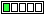All about flooble | fun stuff | Get a free chatterbox | Free JavaScript | Avatarsperplexus dot infoTrig trick (Posted on 2002-11-07)Prove that
sin˛ x + cos˛ x = 1

 See The Solution Submitted by Dulanjana Rating: 2.1818 (11 votes)Comments: ( Back to comment list | You must be logged in to post comments.)Solution| Comment 12 of 13 |Let us consider a right angled triangle ABC with AB, BC and AC
respectively as the altitude, base and the hypotenuse, where
< ABC = 90 degrees.

Then, AB^2 + BC^2 = AC^2
Or, (AB/AC)^2 + (BC/AC)^2 = 1........(*)

We know that:

sin x = altitude/ hypotenuse = AB/ AC
cos x = base/ hypotenuse = BC/AC

Thus, from (*) we obtain:

sin^2(x) + cos^2(x) = 1

 Posted by K Sengupta on 2007-05-28 12:06:41Please log in:

 Search: Search body:
Forums (0)